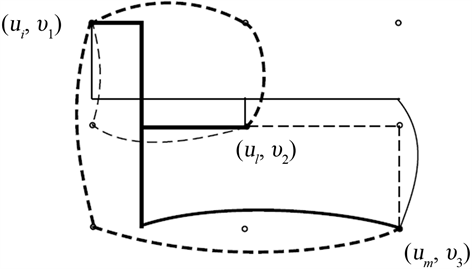# 完全图的笛卡尔积的广义3-连通度The Generalized 3-Connectivity of Cartesian Product of Complete Graphs

DOI: 10.12677/AAM.2019.82036, PDF, HTML, XML, 下载: 428  浏览: 749  国家自然科学基金支持

Abstract: Let S be a set of at least two vertices in a graph G. A subtree T of G is an S-Steiner tree if S⊆V(T). Two S-Steiner trees T1 and T2 are internally disjoint if E(T1)∩E(T2)=∅ and V(T1)∩V(T2)=S. Let KG(S) be the maximum number of internally disjoint S-Steiner trees in G, and let KK(G) be the minimum KG(S) for S ranges over all k-subsets of V(G). In this paper, we study the K3-connectivity of Cartesian product of complete graphs, determine K3(Kn1,Kn2)=n1+n2-3 for any two complete graphs; K3(Kn1,Kn2,...,KnK)=∑i=1kni-K-1 for any k complete graphs, where K≥2.

1. 引言

2. 预备知识

3. 主要定理及其证明

$x=\left({u}_{i},{v}_{1}\right),y=\left({u}_{l},{v}_{2}\right),z=\left({u}_{m},{v}_{3}\right)$${S}^{\prime }=\left\{\left({u}_{c},{v}_{j}\right)|c=i,l,m;j=1,2,3\right\}$ 。考虑如下两种情况。Figure 1. Solid lines in bold for ${T}_{j}$ , $1\le j\le a$ ; Solid lines for ${{T}^{\prime }}_{j}$ , $1\le j\le {n}_{k}-3$ ; Dashed lines for ${{T}^{\prime }}_{{n}_{k}-2}$ ; Dashed lines in bold for ${{T}^{\prime }}_{{n}_{k}-1}$

  Bondy, J.A. and Murty, U.S.R. (2008) Graph Theory, GTM 244. Springer, Berlin.  Chartrand, G., Kapoor, S.F., Lesniak, L. and Lick, D.R. (1984) Generalized Connectivity in Graphs. Bulletin of the Bombay Mathematical Colloquium, 2, 1-6.  Chiue, W.-S. and Shieh, B.-S. (1999) On Connectivity of Cartesian Product of Two Graphs. Applied Mathematics and Computation, 102, 129-137. https://doi.org/10.1016/S0096-3003(98)10041-3  Imrich, W. and Klavžar, S. (2000) Product Graphs. Structure and Recognition. Wiley-Interscience Series in Discrete Mathematics and Optimization, Wiley, New York.  Imrich, W., Klavžar, S. and Rall, D.F. (2008) Topics in Graph Theory. Graphs and Their Cartesian Product. AK Peters, Wellesley.  Klavžar, S. and Spacapan, S. (2008) On the Edge-Connectivity of Cartesian Product Graphs. Asian-European Journal of Mathematics, 1, 93-98.  Sabidussi, G. (1957) Graphs with Given Group and Given Graph-Theoretic Properties. Canadian Journal of Mathematics, 9, 515-525. https://doi.org/10.4153/CJM-1957-060-7  Špacapan, S. (2008) Connectivity of Cartesian Products of Graphs. Applied Mathematics Letters, 21, 682-685. https://doi.org/10.1016/j.aml.2007.06.010  Xu, J. and Yang, C. (2006) Connectivity of Cartesian Product Graphs. Discrete Mathematics, 306, 159-165. https://doi.org/10.1016/j.disc.2005.11.010  Chartrand, G., Okamoto, F. and Zhang, P. (2010) Rainbow Trees in Graphs and Generalized Connectivity. Networks, 55, 360-367.  Li, H. and Wang, J. (2018) The λ3-Connectivity and κ3-Connectivity of Recursive Circulants. Applied Mathematics and Computation, 339, 750-757. https://doi.org/10.1016/j.amc.2018.07.065  Li, H., Li, X. and Sun, Y. (2012) The Generalied 3-Connectivity of Cartesian Product Graphs. Discrete Mathematics and Theoretical Computer Science, 14, 43-54.  Li, S., Li, X. and Zhou, W. (2010) Sharp Bounds for the Generalized Connectivity κ3(G). Discrete Mathematics, 310, 2147-2163. https://doi.org/10.1016/j.disc.2010.04.011  Li, X. and Mao, Y. (2014) The Generalized 3-Connectivity of Lexicographic Product Graphs. Discrete Mathematics and Theoretical Computer Science, 16, 339-354.  Li, X., Mao, Y. and Sun, Y. (2014) On the Generalized (Edge-)Connectivity of Graphs. Australasian Journal of Combinatorics, 58, 304-319.  Li, H., Wu, B., Meng, J. and Ma, Y. (2018) Steiner Tree Packing Number and Tree Connectivity. Discrete Mathematics, 341, 1945-1951. https://doi.org/10.1016/j.disc.2018.03.021  Li, H., Ma, Y., Yang, W. and Wang, Y. (2017) The Generalized 3-Connectivity of Graph Products. Applied Mathematics and Computation, 295, 77-83. https://doi.org/10.1016/j.amc.2016.10.002  Li, X. and Mao, Y. (2016) Generalized Connectivity of Graphs. In: Springer Briefs in Math, Springer, New York. https://doi.org/10.1007/978-3-319-33828-6  Dirac, G.A. (1960) In abstrakten graphen vorhandene voll-standige 4-graphen und ihre unterteilungen. Mathematische Nachrichten, 22, 61-85. https://doi.org/10.1002/mana.19600220107  Lin, S. and Zhang, Q. (2017) The Generalized 4-Connectivity of Hypercubes. Discrete Applied Mathematics, 220, 60-67. https://doi.org/10.1016/j.dam.2016.12.003